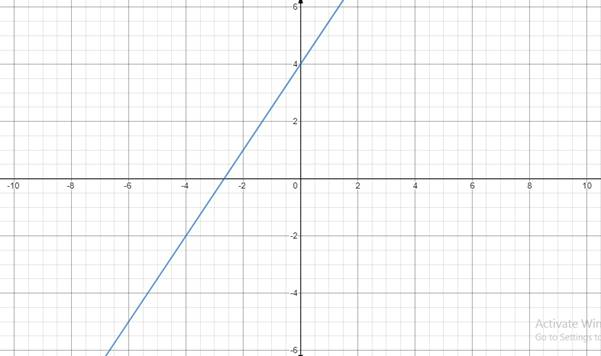# All the real solution of equation.### Precalculus: Mathematics for Calcu...

6th Edition
Stewart + 5 others
Publisher: Cengage Learning
ISBN: 9780840068071### Precalculus: Mathematics for Calcu...

6th Edition
Stewart + 5 others
Publisher: Cengage Learning
ISBN: 9780840068071

#### Solutions

Chapter 1.10, Problem 39E
To determine

## All the real solution of equation.

Expert Solution

Equation of the line 3x2y8=0 .

### Explanation of Solution

Given information:

slope=32point=(2,1)

Formula used:

(yy1)=m(xx1)

a)b) Equation of line:

(yy1)=m(xx1)

Now putting the value of slope and points in above equation.

(y1)=32(x+2)2(y1)=3x+62y2=3x+63x2y8=0

Equation of the line passing through (2,1) with slope 32 is 3x2y8=0

### Have a homework question?

Subscribe to bartleby learn! Ask subject matter experts 30 homework questions each month. Plus, you’ll have access to millions of step-by-step textbook answers!